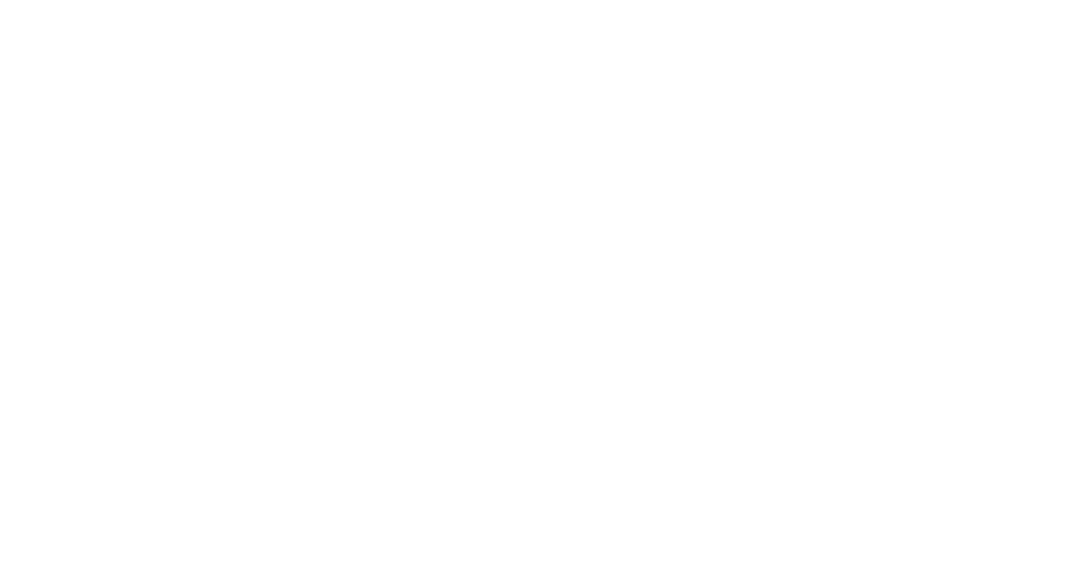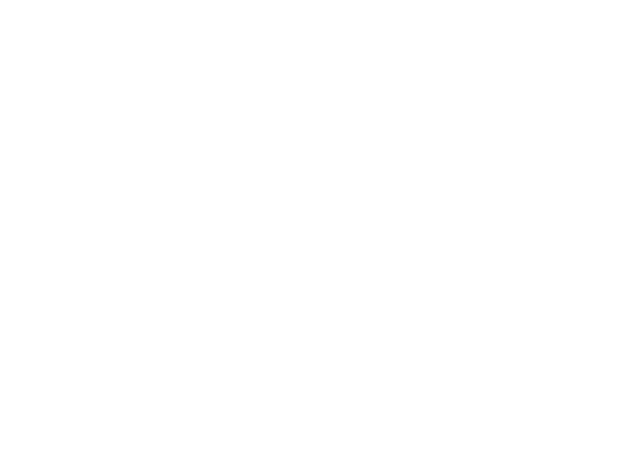但很可惜的是，训练数据和秘笈还有一个特点很相似，那就是可遇而不可求！也就是说很难获取，除了那些公共数据集之外，如果用户想基于自己的业务场景准备数据的话，不仅数据的生产和标注过程会比较复杂，而且一般需要的数量规模也会非常庞大，因为只有充足的数据，才能确保模型训练的效果，这导致数据集的制作成本往往非常高。这个情况在计算机视觉领域尤甚，因为图像要一张一张拍摄与标注，要是搞个几十万图片，想想都让人“不寒而栗”！

#### 8大数据增广方法

1. 图像解码，也就是将图像转为Numpy格式的数据，简写为 ImageDecode。

1. 图像随机裁剪，随机将图像的长宽均裁剪为 224 大小，简写为 RandCrop。

1. 水平方向随机翻转，简写为 RandFlip。

1. 图像数据的归一化，简写为 Normalize。

1. 图像数据的重排。图像的数据格式为[H, W, C]（即高度、宽度和通道数），而神经网络使用的训练数据的格式为[C, H, W]，因此需要对图像数据重新排列，例如[224, 224, 3] 变为 [3, 224, 224]，简写为 Transpose。

1. 多幅图像数据组成 batch ，如 BatchSize 个 [3, 224, 224] 的图像数据拼组成 [batch-size, 3, 224, 224]，简写为 Batch。

1. 图像变换类：对 RandCrop 后的 224 的图像进行一些变换，包括AutoAugment和RandAugment。

1. 图像混叠：对 Batch 后的数据进行混合或叠加，包括Mixup和Cutmix。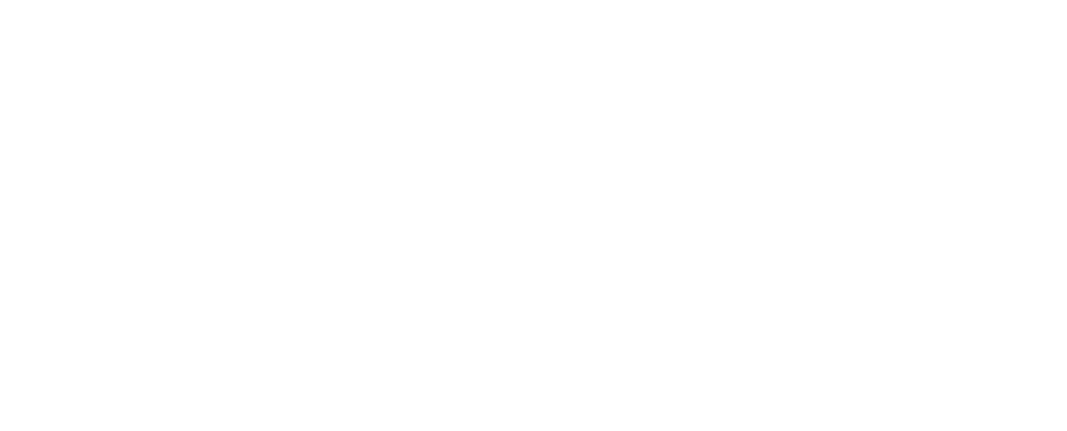图像变换类

0 1

#### AutoAugment

https://arxiv.org/abs/1805.09501v1

```from ppcls.data.imaug import DecodeImage
from ppcls.data.imaug import ResizeImage
from ppcls.data.imaug import ImageNetPolicy
from ppcls.data.imaug import transform
size = 224
# 图像解码
decode_op = DecodeImage()
# 图像随机裁剪
resize_op = ResizeImage(size=(size, size))
# 使用AutoAugment图像增广方法
autoaugment_op = ImageNetPolicy()
ops = [decode_op, resize_op, autoaugment_op]
# 图像路径
imgs_dir = “/imgdir/xxx.jpg”
fnames = os.listdir(imgs_dir)
for f in fnames:
img = transform(data, ops)```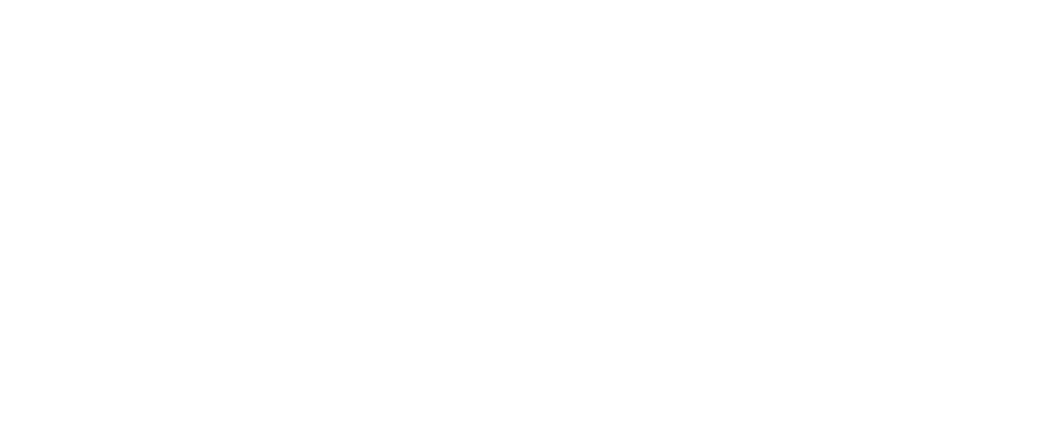#### RandAugment

https://arxiv.org/pdf/1909.13719.pdf

AutoAugment 的搜索方法比较暴力，直接在数据集上搜索针对该数据集的最优策略，计算量会很大。在 RandAugment对应的论文中作者发现，针对越大的模型，越大的数据集，使用 AutoAugment 方式搜索到的增广方式产生的收益也就越小；而且这种搜索出的最优策略是针对指定数据集的，迁移能力较差，并不太适合迁移到其他数据集上。

```from ppcls.data.imaug import DecodeImage
from ppcls.data.imaug import ResizeImage
from ppcls.data.imaug import RandAugment
from ppcls.data.imaug import transform
size = 224
# 图像解码
decode_op = DecodeImage()
# 图像随机裁剪
resize_op = ResizeImage(size=(size, size))
# 使用RandAugment图像增广方法
randaugment_op = RandAugment()
ops = [decode_op, resize_op, randaugment_op]
# 图像路径
imgs_dir = “/imgdir/xxx.jpg”
fnames = os.listdir(imgs_dir)
for f in fnames:
img = transform(data, ops)```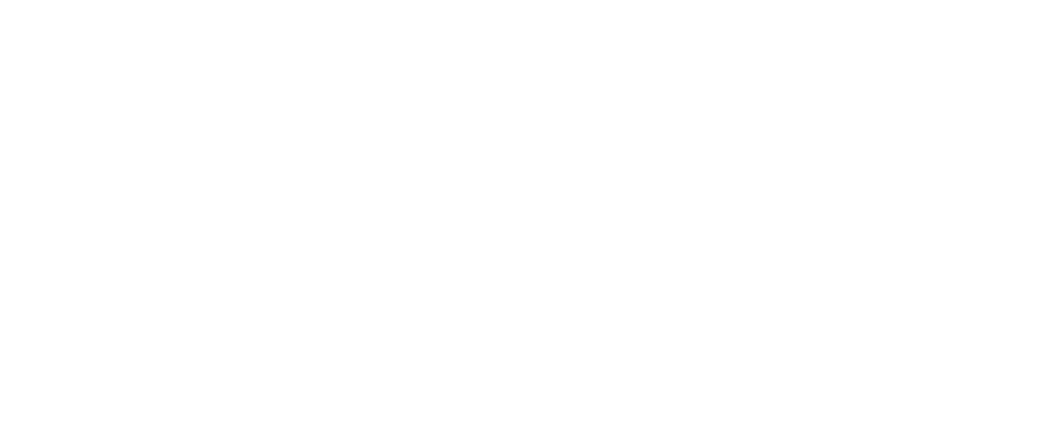#### Cutout

https://arxiv.org/abs/1708.04552

Cutout 可以理解为Dropout的一种扩展操作，不同的是Dropout是对图像经过网络后生成的特征进行遮挡，而 Cutout 是直接对输入的图像进行遮挡，相对于Dropout对噪声的鲁棒性更好。作者在论文中也进行了说明，这样做法有以下两点优势：

```from ppcls.data.imaug import DecodeImage
from ppcls.data.imaug import ResizeImage
from ppcls.data.imaug import Cutout
from ppcls.data.imaug import transform
size = 224
# 图像解码
decode_op = DecodeImage()
# 图像随机裁剪
resize_op = ResizeImage(size=(size, size))
# 使用Cutout图像增广方法
cutout_op = Cutout(n_holes=1, length=112)
ops = [decode_op, resize_op, cutout_op]
# 图像路径
imgs_dir = “/imgdir/xxx.jpg”
fnames = os.listdir(imgs_dir)
for f in fnames:
img = transform(data, ops)```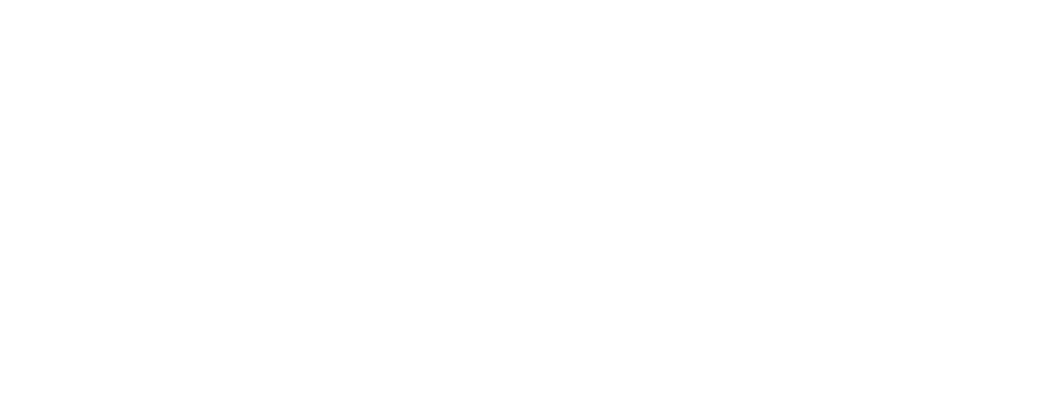#### RandomErasing

https://arxiv.org/pdf/1708.04896.pdf

RandomErasing 与 Cutout 方法类似，同样是为了解决训练出的模型在有遮挡数据上泛化能力较差的问题，作者在论文中也指出，随机裁剪的方式与随机水平翻转具有一定的互补性。作者也在行人再识别（REID）上验证了该方法的有效性。与Cutout不同的是，在RandomErasing中，图片以一定的概率接受该种预处理方法，生成掩码的尺寸大小与长宽比也是根据预设的超参数随机生成。

```from ppcls.data.imaug import DecodeImage
from ppcls.data.imaug import ResizeImage
from ppcls.data.imaug import ToCHWImage
from ppcls.data.imaug import RandomErasing
from ppcls.data.imaug import transform
size = 224
# 图像解码
decode_op = DecodeImage()
# 图像随机裁剪
resize_op = ResizeImage(size=(size, size))
# 使用RandomErasing图像增广方法
randomerasing_op = RandomErasing()
ops = [decode_op, resize_op, tochw_op, randomerasing_op]
# 图像路径
imgs_dir = “/imgdir/xxx.jpg”
fnames = os.listdir(imgs_dir)
for f in fnames:
img = transform(data, ops)
img = img.transpose((1, 2, 0))```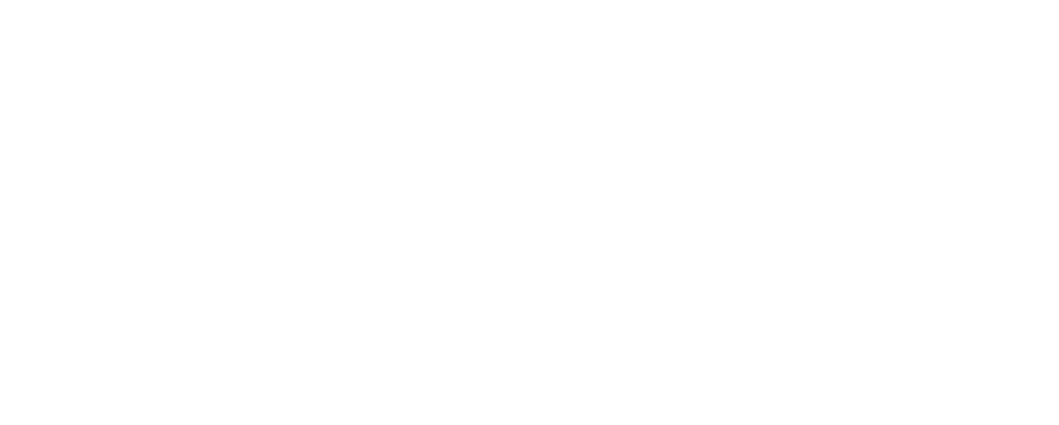#### HideAndSeek

https://arxiv.org/pdf/1811.02545.pdf

HideAndSeek方法将图像分为若干大小相同的区域块(patch)，对于每块区域，都以一定的概率生成掩码，如下图所示，可能是完全遮挡、完全不遮挡或者遮挡部分。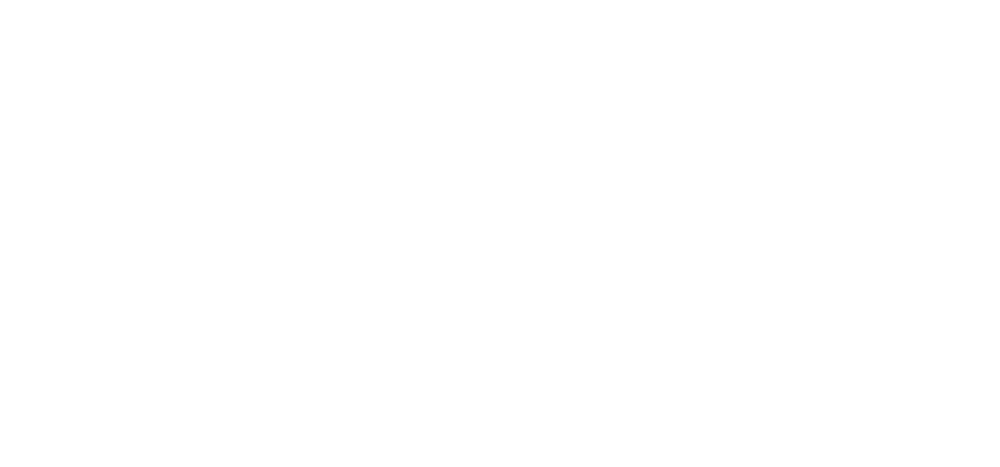```from ppcls.data.imaug import DecodeImage
from ppcls.data.imaug import ResizeImage
from ppcls.data.imaug import ToCHWImage
from ppcls.data.imaug import HideAndSeek
from ppcls.data.imaug import transform
size = 224
# 图像解码
decode_op = DecodeImage()
# 图像随机裁剪
resize_op = ResizeImage(size=(size, size))
# 使用HideAndSeek图像增广方法
hide_and_seek_op = HideAndSeek()
ops = [decode_op, resize_op, tochw_op, hide_and_seek_op]
# 图像路径
imgs_dir = “/imgdir/xxx.jpg”
fnames = os.listdir(imgs_dir)
for f in fnames:
img = transform(data, ops)
img = img.transpose((1, 2, 0))```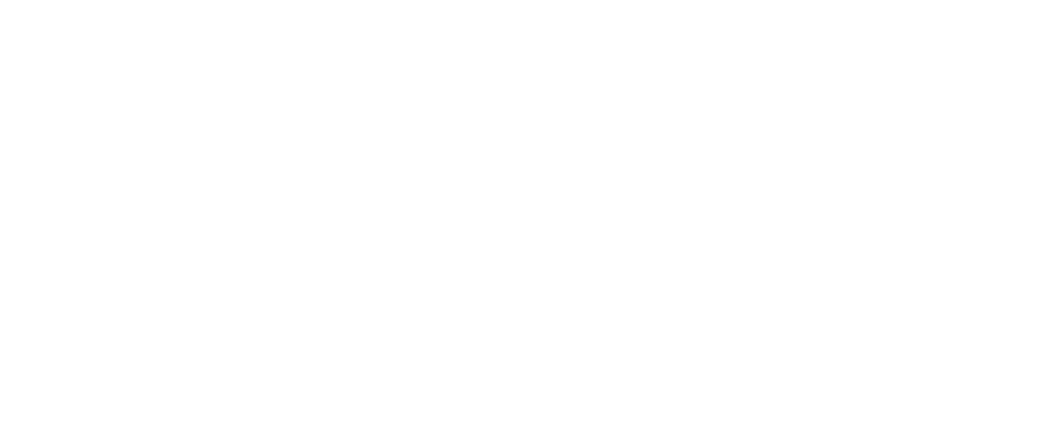#### 06

https://arxiv.org/abs/2001.04086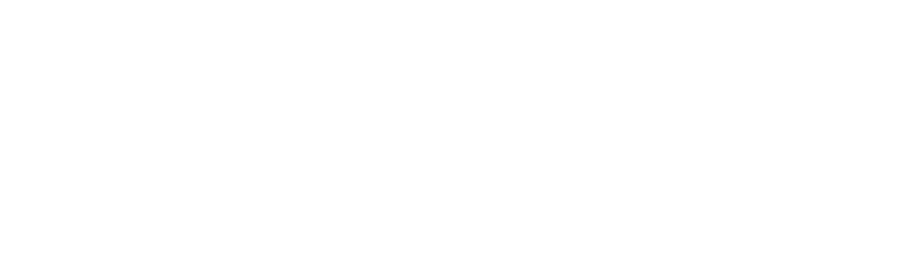```from data.imaug import DecodeImage
from data.imaug import ResizeImage
from data.imaug import ToCHWImage
from data.imaug import transform
size = 224
# 图像解码
decode_op = DecodeImage()
# 图像随机裁剪
resize_op = ResizeImage(size=(size, size))
# 图像数据的重排
tochw_op = ToCHWImage()
ops = [decode_op, resize_op, tochw_op, gridmask_op]
# 图像路径
imgs_dir = “/imgdir/xxx.jpg”
fnames = os.listdir(imgs_dir)
for f in fnames:
img = transform(data, ops)
img = img.transpose((1, 2, 0))```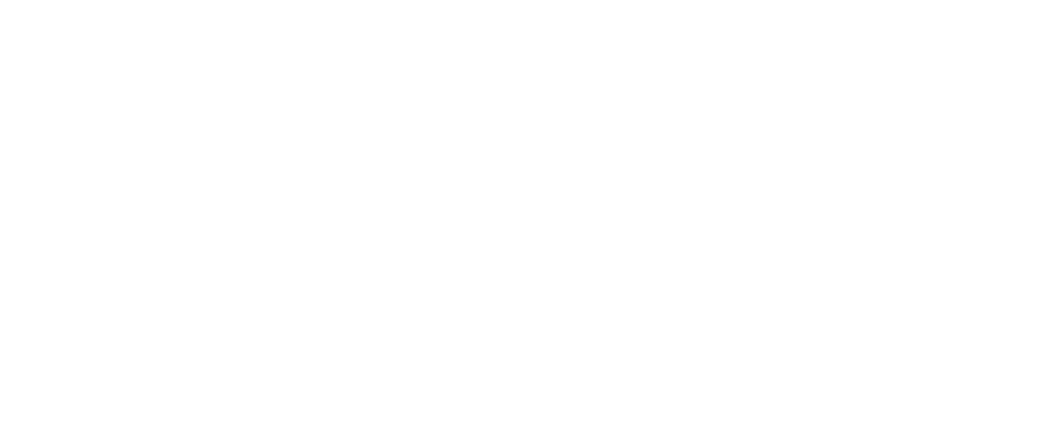#### Mixup

https://arxiv.org/pdf/1710.09412.pdf

Mixup是最先提出的图像混叠增广方案，其原理就是直接对两幅图的像素以一个随机的比例进行相加，不仅简单，而且方便实现，在图像分类和目标检测领域上都取得了不错的效果。为了便于实现，通常只对一个 batch 内的数据进行混叠，在Cutmix中也是如此。

```from ppcls.data.imaug import DecodeImage
from ppcls.data.imaug import ResizeImage
from ppcls.data.imaug import ToCHWImage
from ppcls.data.imaug import transform
from ppcls.data.imaug import MixupOperator
size = 224
# 图像解码
decode_op = DecodeImage()
# 图像随机裁剪
resize_op = ResizeImage(size=(size, size))
# 图像数据的重排
tochw_op = ToCHWImage()
# 使用HideAndSeek图像增广方法
hide_and_seek_op = HideAndSeek()
# 使用Mixup图像增广方法
mixup_op = MixupOperator()
ops = [decode_op, resize_op, tochw_op]
imgs_dir = “/imgdir/xxx.jpg”  #图像路径
batch = []
fnames = os.listdir(imgs_dir)
for idx, f in enumerate(fnames):
img = transform(data, ops)
batch.append( (img, idx) ) # fake label
new_batch = mixup_op(batch)```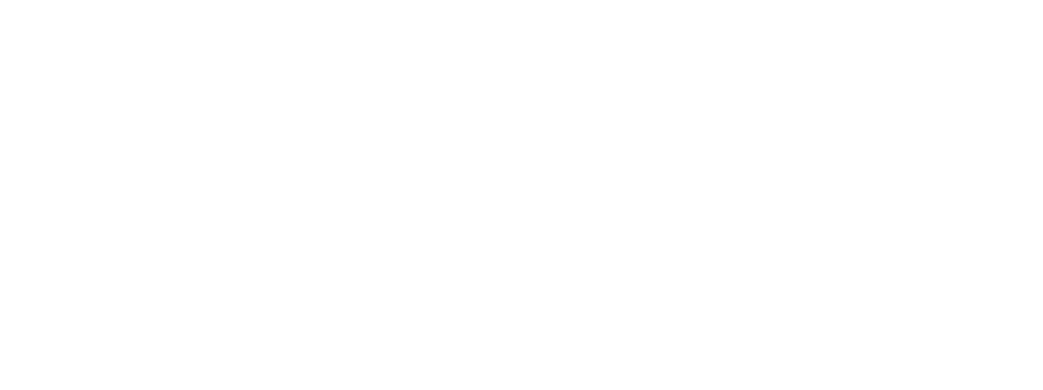#### Cutmix

https://arxiv.org/pdf/1905.04899v2.pdf

```from ppcls.data.imaug import DecodeImage
from ppcls.data.imaug import ResizeImage
from ppcls.data.imaug import ToCHWImage
from ppcls.data.imaug import transform
from ppcls.data.imaug import CutmixOperator
size = 224
# 图像解码
decode_op = DecodeImage()
# 图像随机裁剪
resize_op = ResizeImage(size=(size, size))
# 图像数据的重排
tochw_op = ToCHWImage()
# 使用HideAndSeek图像增广方法
hide_and_seek_op = HideAndSeek()
# 使用Cutmix图像增广方法
cutmix_op = CutmixOperator()

ops = [decode_op, resize_op, tochw_op]
imgs_dir = “/imgdir/xxx.jpg”  #图像路径
batch = []
fnames = os.listdir(imgs_dir)
for idx, f in enumerate(fnames):
img = transform(data, ops)
batch.append( (img, idx) ) # fake label
new_batch = cutmix_op(batch)```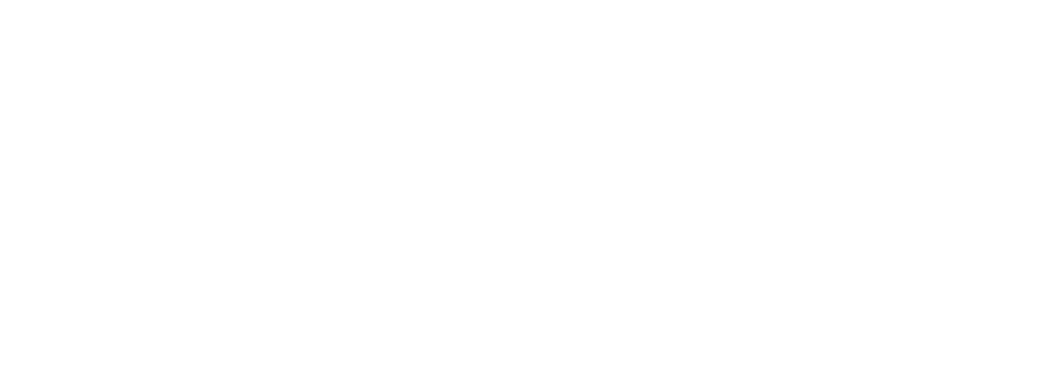#### 实验